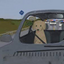Ask your own question, for FREE!
Mathematicskiller:

Solve the inequality. Graph the solution. 3p+2≥−10 The solution isjhonyy9:

like a first step subtract from both sides 2 so what will get ?jhonyy9:

@supie any idea ?supie:

So when i subtract 2 from both sides i got $$3p≥−12$$ because $$3p+2−2≥−10−2$$ -10-2=12 so $$3p≥−12$$ then divide both sides by 3 ???jhonyy9:

$$\color{#0cbb34}{\text{Originally Posted by}}$$ @supie So when i subtract 2 from both sides i got $$3p≥−12$$ because $$3p+2−2≥−10−2$$ -10-2=12 so $$3p≥−12$$ then divide both sides by 3 ??? $$\color{#0cbb34}{\text{End of Quote}}$$ good ideajhonyy9:

continue pls.jhonyy9:

@killer ok ?supie:

Ok so $$\LARGE\frac{3p}{3}≥\frac{-12}{3}$$ $$\cancel{\frac{3p}{p}}≥\frac{-12}{3}$$ (3p/p cancels) so all that is lef tis $$\frac{-12}{3}$$ so that needs to be simplified $$\frac{-12}{3}=?$$ $$p≥?$$supie:

left is * ._.jhonyy9:

$$\color{#0cbb34}{\text{Originally Posted by}}$$ @supie Ok so $$\LARGE\frac{3p}{3}≥\frac{-12}{3}$$ $$\cancel{\frac{3p}{p}}≥\frac{-12}{3}$$ (3p/p cancels) so all that is lef tis $$\frac{-12}{3}$$ so that needs to be simplified $$\frac{-12}{3}=?$$ $$p≥?$$ $$\color{#0cbb34}{\text{End of Quote}}$$ attention pls on the left side 3 cancel by 3 not p by pjhonyy9:

3p/3 not 3p/psupie:

Oh that's what I meant.jhonyy9:

$$\color{#0cbb34}{\text{Originally Posted by}}$$ @jhonyy9 $$\color{#0cbb34}{\text{Originally Posted by}}$$ @supie Ok so $$\LARGE\frac{3p}{3}≥\frac{-12}{3}$$ $$\cancel{\frac{3p}{p}}≥\frac{-12}{3}$$ (3p/p cancels) so all that is lef tis $$\frac{-12}{3}$$ so that needs to be simplified $$\frac{-12}{3}=?$$ $$p≥?$$ $$\color{#0cbb34}{\text{End of Quote}}$$ attention pls on the left side 3 cancel by 3 not p by p $$\color{#0cbb34}{\text{End of Quote}}$$ on the left side first you ve wrote 3p/3 but you continue with 3p/pjhonyy9:

@supiesupie:

Yes i know. I meant $$\frac{3p}{3}$$ but I accidently did $$\frac{3p}{p}$$jhonyy9:

$$\color{#0cbb34}{\text{Originally Posted by}}$$ @jhonyy9 $$\color{#0cbb34}{\text{Originally Posted by}}$$ @jhonyy9 $$\color{#0cbb34}{\text{Originally Posted by}}$$ @supie Ok so $$\LARGE\frac{3p}{3}≥\frac{-12}{3}$$ $$\cancel{\frac{3p}{p}}≥\frac{-12}{3}$$ (3p/p cancels) so all that is lef tis $$\frac{-12}{3}$$ so that needs to be simplified $$\frac{-12}{3}=?$$ $$p≥?$$ $$\color{#0cbb34}{\text{End of Quote}}$$ attention pls on the left side 3 cancel by 3 not p by p $$\color{#0cbb34}{\text{End of Quote}}$$ on the left side first you ve wrote 3p/3 but you continue with 3p/p $$\color{#0cbb34}{\text{End of Quote}}$$ @killer did you get the answer now ?jhonyy9:

questions ?jhonyy9:

@supie how you graph the solution ?

Can't find your answer? Make a FREE account and ask your own questions, OR help others and earn volunteer hours!

Join our real-time social learning platform and learn together with your friends!
Latest Questionsvybez: The eleven states that seceded from the Union in 1860-61 formed a new political entity called the Choose 1 answer: (Choice A) A Articles of Confederation.
8 seconds ago 3 Replies 0 MedalsBabygirlella06: Prime Minister Nehru wanted to avoid the Cold War by not siding with either the USA or the USSR.
55 seconds ago 1 Reply 0 Medalskatelyn865: whats the coolst thing you evey seen
8 minutes ago 9 Replies 2 Medalsvybez: help ;o
16 minutes ago 19 Replies 1 Medalkristiannnnnt: What's your average WPM?
33 minutes ago 0 Replies 0 MedalsSingleGirl123: What inequality does the number line graph represent?
22 minutes ago 8 Replies 0 Medalskristiannnnnt: RATE
29 minutes ago 13 Replies 1 MedalForeverangel: What does the number next to the element name indicate?
58 minutes ago 5 Replies 0 Medalsfernandez06: HELP Point D is the center of the inscribed circle of ABC. Which step can you use to draw the inscribed circle of ABC? A.
1 hour ago 0 Replies 0 Medalsfernandez06: Point D is the center of the inscribed circle of ABC. Which step can you use to draw the inscribed circle of ABC? A.
1 hour ago 0 Replies 0 Medals
Can't find your answer? Make a FREE account and ask your own questions, OR help others and earn volunteer hours!

Join our real-time social learning platform and learn together with your friends!